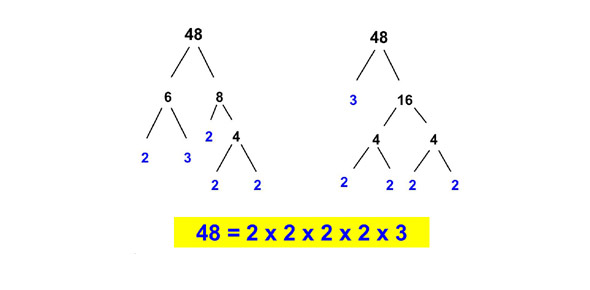# Factors And Factoring

7 Questions | Total Attempts: 23SettingsIn this quiz you will be taking is based on Factors and Factoring. Visit www. Mathisfun101. Webs. Com if you need any help!

Related Topics
• 1.
In 20 words or more explain what factors are, how you can use them and how to find factors of a number!
• 2.
A factor is the answer to a multiplication question?
• A.

True

• B.

False

• 3.
A _________ is the result of two factors being multiplied.
• 4.
With factors you can only...
• A.

• B.

Multiply

• C.

Divide

• 5.
In the following equation, what are the factors?2 x 4 x 5(2=80
• A.

2,4,5,80

• B.

2,5,4,2

• C.

1,80,55,4

• 6.
What is the opposite of expanding?
• A.

Multiply

• B.

Dividing

• C.

Factoring

• 7.
Factoring is when you try to figure out what to multiply to get an expression then adding the expression. Right?
• A.

True

• B.

False